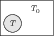# Kirchhoff's Law

## Problems from IIT JEE

Problem (IIT JEE 2006):In a dark room with ambient temperature $T_0$, a black body is kept at temperature $T.$ Keeping the temperature of the black body constant (at $T$), sun rays are allowed to fall on the black body through a hole in the roof of the dark room. Assuming that there is no change in the ambient temperature of the room, which of the following statement(s) is (are) correct?

1. The quantity of radiation absorbed by the black body in unit time will increase.
2. Since emissivity $=$ absorptivity, hence the quantity of radiation emitted by black body in unit time will increase.
3. Black body radiates more energy in unit time in the visible spectrum.
4. The reflected energy in unit time by the black body remains same.

Solution: When sun rays fall on a black body, a portion of incident photons are absorbed and the remaining are reflected. So, the net quantity of radiations absorbed by the black body in unit time increases. At the same time, since emissivity and absorptivity are equal, the quantity of radiations emitted by the black body in unit time also increases. The wavelength $\lambda_\text{max}$ at which maximum radiation takes place depends on black body temperature $T$ and is given by Wien's displacement law $\lambda_\text{max}T=b$. Thus, A and B are correct.

Problem (IIT JEE 2003):The temperature ($T$) \emph{versus} time ($t$) graphs of two bodies X and Y with equal surface areas are shown in the figure. If the emissivity and the absorptivity of X and Y are $E_x$, $E_y$ and $a_x$, $a_y$, respectively, then,

1. $E_x > E_y$ and $a_x < a_y$
2. $E_x < E_y$ and $a_x > a_y$
3. $E_x > E_y$ and $a_x > a_y$
4. $E_x < E_y$ and $a_x < a_y$

Solution: The rate of cooling for $x$ is greater than that of $y$. The cooling occurs due to heat loss by radiation. By Stefan's law, the rate of heat loss is, \begin{align} \mathrm{d}Q/\mathrm{d}t=\sigma EA(T^4-T_0^4),\nonumber \end{align} where $E$ is emissivity. Hence, $E_x > E_y$. Since good emitters are good absorbers, the absorptivity $a_x > a_y$.